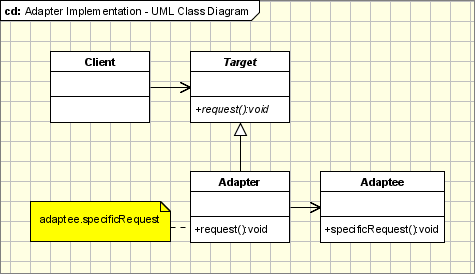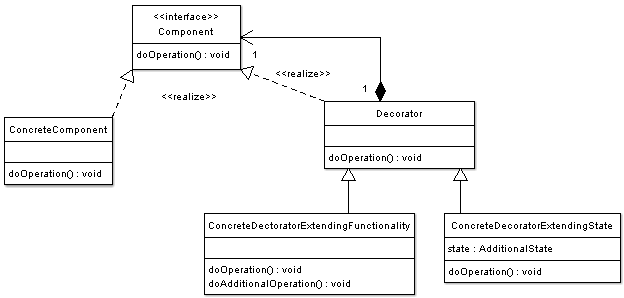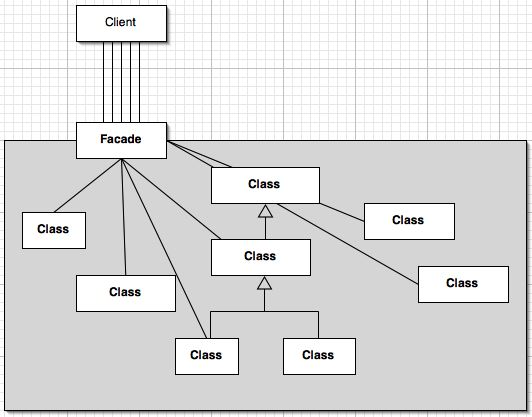# Introduction

Whilst Creational patterns allow for high degrees of flexibility in the instantiation of objects, Structural patterns provide flexibility with the composition of objects to form larger, complex objects. This is achieved through the use of inheritance and composition. Structural patterns describe ways to compose objects to allow for new functionality at run-time, which is impossible with static class construction.

As with the Creational patterns, we will consider only a subset of the Structural patterns. There are many more Structural patterns to learn, and it is recommended that you review the remaining patterns.

## Purpose

An adaptor is used to convert the interface of classes into an expected, standardized interface. The Adaptor pattern allows compatibility between objects and client code.

## Description

Sometimes we have the situation where a level of abstraction is required between client code and a number of different services, technologies or other classes. For example, if we want to create a Message class, and abstract away from the various technologies with which we can create or receive a message (ie: email, instant messenger, SMS, Twitter, Facebook, etc.), we could use the Abstract pattern to accomplish this. We might have classes for the API's of each of the various technologies with which to send and receive messages, and apply the adaptor to enable the client code to use a single, standardized interface for sending and receiving messages, irrespective of the technology used.

## Solution

The Adaptor design pattern has the following components:

• Target: The abstracted interface used by the Client.
• Client: The client code making use of the Adaptee's functionality via the Adaptor.
• Adaptee: The class being adapted by the Adaptor for use by the Client through the Target.
• Adaptor: The class which provides the standardized interface to the functionality of the Adaptee.

The Client makes a request of the Target, who, in turn, makes a request of the Adaptor, which finally makes a specific request of the Adaptee which is being standardized by the Adaptor.## Python Implementation

To learn how to apply the Adaptor pattern in Python, let us have a look at the above Messenger example implemented in Python code.

``````from abc import ABCMeta, abstractmethod

class Message:
__metaclass__ = ABCMeta

@staticmethod
def Factory():

@abstractmethod
def send(self, method, to, message):
pass

@abstractmethod
pass

def send(self, method, to, message):
if method == "email":
else:
raise Exception("Invalid method.")

if method == "email":
else:
raise Exception("Invalid method.")

class Email:

def send_email(self, to, message):
print "Sending an email to %s" % (to)
# Code to send an email goes here
pass

print "Receiving an email"
# Code to receive an email
pass

def send_fb_message(self, to, message):
print "Sending a Facebook message to %s" % (to)
# Code to send a Facebook message
pass

pass

if __name__ == '__main__':
messager = Message.Factory()
messager.send("email", "someone@domain.com", "Hi. How are you today?")
``````

In the above example, the client code at the bottom (if __name__ == '__main__':) calls the Target, Message() which makes use of the Adaptor, MessageAdaptor() to provide a standardized interface for sending and receiving messages independently of the specific technology or protocol used.

# Decorator

## Purpose

Automatically extend functionality as an alternative to inheritance. The decorator intercepts messages between two objects and provides the extended functionality seamlessly.

## Description

Let's imagine that we have a class which handles comments for a website. Rather than worrying about converting the comment into HTML within the Comment() class, we might want to apply a Decorator to handle the formatting automatically. Thus, as the Comment() object returns the comment to the client code, the Decorator would intercept the message, and apply the HTML formatting.

## Solution

The Decorator design pattern is comprised of the following elements:

• Component: Defines the abstract common interface for objects to be extended by Decorators.
• Concrete Component: This is the class for objects which can be extended by Decorators, inheriting from the Component class.
• Decorator: Abstract class which contains a reference to the Component class.
• Concrete Decorator: Contains the code to extend the functionality of the Concrete Component.Note: If there is only a need for a single piece of functionality to be extended, there is no need to make use of the abstract Decorator class and just define the Concrete Decorator.

## Python Implementation

In Python, we have a specific syntax for Decorators. You will have seen in earlier sections something like

``````@staticmethod
``````

Which is an example of a Decorator which converts the class method into a static method. Note the "@" prefix. Let's look at an example of using a Decorator in Python.

``````def htmlify(Component):
def ConcreteDecorator(*obj, **kwargs):
html = obj.comment
html = html.replace("\n", "")
html = html.replace("\t", "    ")
html = html.replace(" ", " ")
return html
return ConcreteDecorator

class Comment:

comment = ""

def set_comment(self, comment):
self.comment = comment

@htmlify
def get_comment(self):
return self.comment

if __name__ == "__main__":
my_comment = Comment()
my_comment.set_comment("Hi,\nThis is my comment.")
print my_comment.get_comment()
``````

Here, we define the decorator first, htmlify, and then we prefix the get_comment() method in the Comment() class with the decorator syntax:

``````@htmlify
``````

This causes the instance of the function at run time to be passed to the decorator, which then modifies the comment variable, and returns its value.

## Purpose

Provide a single, unified interface for a collection of classes or a sub-system. The Facade design pattern simplifies usage of the sub-system through a single, simplified interface in a single Facade class.

## Description

It is a common development practice to develop systems in the form of sub-systems or modules. An important goal when developing with sub-systems is to minimize the inter-module communication. By using the Facade design pattern, we achieve this purpose by having a single point for communication.

## Solution

The Facade design pattern has the following components:

• Facade: Delegates the requests made by the Client code to the appropriate Subsystem Classes.
• Subsystem Classes: Collection of classes which implement the functionality of the subsystem.
• Client: The client code utilizing Subsystem functionality via the Facade.## Python Implementation

To demonstrate the Facade design pattern in Python, let's consider an example where we have a submodule which provides the Statistics functionality. We will then define a StatisticsFacade class to simplify interaction between the client code and the Statistics module.

``````import math

class Stats_NormalDistribution:

mean = 0
standard_deviation = 0

def __init__(self, mean, sd):
self.mean = mean
self.standard_deviation = sd

def graph(self):
# Code to draw graph
pass

class Stats_StraightLine:

intersection = 0

def __init__(self, m, c):
self.intersection = c

def graph(self):
# Code to draw graph
pass

class Stats_Calculations:

def average(self, x_arr):
total = 0
for i in x_arr:
total = total + i
average = total / len(x_arr)
return average

def standard_deviation(self, x_arr):
average = self.average(x_arr)
sum = 0
for i in x_arr:
sum = sum + ((i - average) ** 2)
sqr_avg = sum / len(x_arr)
sd = math.sqrt(sqr_avg)
return sd

normal = 0
straight_line = 0
calculations = 0

def __init__(self):
self.normal = Stats_NormalDistribution(0, 0)
self.straight_line = Stats_StraightLine(0, 0)
self.calculations = Stats_Calculations()

def average(self, x_arr):
return self.calculations.average(x_arr)

def standard_deviation(self, x_arr):
return self.calculations.standard_deviation(x_arr)

def normal_graph(self, avg, mean):
self.normal.average = avg
self.normal.mean = mean
return self.normal.graph()

self.straight_line.intersection = intersection
return self.straight_line.graph()

if __name__ == "__main__":
x = [1, 4, 6, 12, 13, 4, 19, 19, 20, 21, 22, 23, 31, 40, 45]
avg = stats.average(x)
sd = stats.standard_deviation(x)
print x
print "Average = %d, Standard Deviation = %d" % (avg, sd)
normal_graph = stats.normal_graph(avg, sd)
intersection = 1
``````

# Exercises

Give three scenarios where it would be appropriate to utilize the following design patterns: